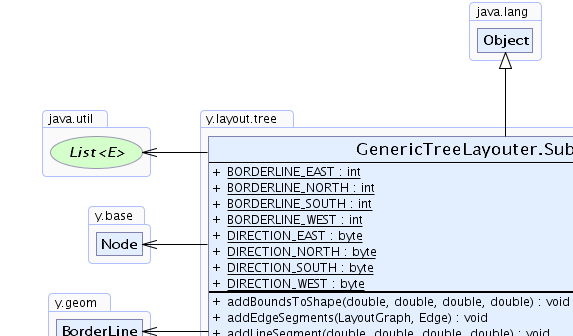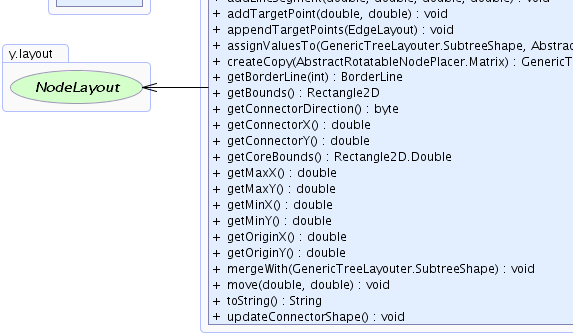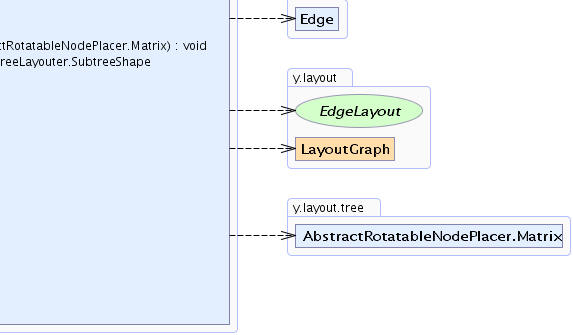Search this API

## y.layout.tree Class GenericTreeLayouter.SubtreeShape

```java.lang.Objecty.layout.tree.GenericTreeLayouter.SubtreeShape
```
Enclosing class:
GenericTreeLayouter

`public static final class GenericTreeLayouter.SubtreeShapeextends java.lang.Object`

A `GenericTreeLayouter.SubtreeShape` represents the position and the borders of a subtree. `NodePlacer`s will move `GenericTreeLayouter.SubtreeShape`s and use the borders to calculate distances between the subtrees. When two subtrees are placed in relation to their common root, their `GenericTreeLayouter.SubtreeShape`s are merged to represent the new subtree.

The borders on each side of the `GenericTreeLayouter.SubtreeShape` are modeled with `BorderLine`s. The resulting area includes the borders of each node and edge in the corresponding subtree. It can also contain the bounds of labels belonging to those nodes and edges.

The `GenericTreeLayouter.SubtreeShape` also describes a connector defining the location to which the edge from its parent is attached. It is possible to add some bends (target points) at the end of this edge and include them in the `GenericTreeLayouter.SubtreeShape`. During layout calculation, the last target point is the connection point for the edge.

`getBorderLine(int)`, `getConnectorX()`, `getConnectorY()`, `addBoundsToShape(double, double, double, double)`, `addTargetPoint(double, double)`Field Summary
`static int` `BORDERLINE_EAST`
Side constant that defines the index of the eastern border line.
`static int` `BORDERLINE_NORTH`
Side constant that defines the index of the northern border line.
`static int` `BORDERLINE_SOUTH`
Side constant that defines the index of the southern border line.
`static int` `BORDERLINE_WEST`
Side constant that defines the index of the western border line.
`static byte` `DIRECTION_EAST`
A direction constant that describes that the last connector segment points to the right.
`static byte` `DIRECTION_NORTH`
A direction constant that describes that the last connector segment points up.
`static byte` `DIRECTION_SOUTH`
A direction constant that describes that the last connector segment points down.
`static byte` `DIRECTION_WEST`
A direction constant that describes that the last connector segment points to the left.

Method Summary
` void` ```addBoundsToShape(double x, double y, double width, double height)```
Extends this `GenericTreeLayouter.SubtreeShape` instance by the given rectangle.
` void` ```addEdgeSegments(LayoutGraph graph, Edge edge)```
Adds all edge segments of the given edge to this `GenericTreeLayouter.SubtreeShape` instance.
` void` ```addLineSegment(double x1, double y1, double x2, double y2)```
Extends this `GenericTreeLayouter.SubtreeShape` instance by the given segment.
` void` ```addTargetPoint(double x, double y)```
Adds another point to the connector.
` void` `appendTargetPoints(EdgeLayout edgeLayout)`
Appends all target points that have previously been added to this shape to the given `EdgeLayout` instance.
` void` ```assignValuesTo(GenericTreeLayouter.SubtreeShape toShape, AbstractRotatableNodePlacer.Matrix modificationMatrix)```
Copies the state of this `GenericTreeLayouter.SubtreeShape` instance to the given shape applying a modification matrix.
` GenericTreeLayouter.SubtreeShape` `createCopy(AbstractRotatableNodePlacer.Matrix matrix)`
Creates a clone of this `GenericTreeLayouter.SubtreeShape` instance, modifying it using the provided modification matrix.
` BorderLine` `getBorderLine(int index)`
Returns the `BorderLine` instance that describes the `GenericTreeLayouter.SubtreeShape` at the given side.
` java.awt.geom.Rectangle2D` `getBounds()`
Returns the current bounds of this `GenericTreeLayouter.SubtreeShape` instance.
` byte` `getConnectorDirection()`
Returns the direction of the last connector segment.
` double` `getConnectorX()`
Returns the current x-coordinate of the connection point to which the layout algorithm connects the ingoing edge.
` double` `getConnectorY()`
Returns the current y-coordinate of the connection point to which the layout algorithm connects the ingoing edge.
` java.awt.geom.Rectangle2D.Double` `getCoreBounds()`
Returns the bounds of the `NodeLayout` of the root node of this `GenericTreeLayouter.SubtreeShape` instance.
` double` `getMaxX()`
Returns the current maximum x-coordinate of this `GenericTreeLayouter.SubtreeShape` instance.
` double` `getMaxY()`
Returns the current maximum y-coordinate of this `GenericTreeLayouter.SubtreeShape` instance.
` double` `getMinX()`
Returns the current minimum x-coordinate of this `GenericTreeLayouter.SubtreeShape` instance.
` double` `getMinY()`
Returns the current minimum y-coordinate of this `GenericTreeLayouter.SubtreeShape` instance.
` double` `getOriginX()`
Returns the current x-coordinate of the origin of this `GenericTreeLayouter.SubtreeShape` instance.
` double` `getOriginY()`
Returns the current y-coordinate of the origin of this `GenericTreeLayouter.SubtreeShape` instance.
` void` `mergeWith(GenericTreeLayouter.SubtreeShape other)`
Merges the given `GenericTreeLayouter.SubtreeShape` instance with this `GenericTreeLayouter.SubtreeShape` instance.
` void` ```move(double dx, double dy)```
Moves this `GenericTreeLayouter.SubtreeShape` instance and its connector by the given offsets.
` java.lang.String` `toString()`
Returns a string representation of the `GenericTreeLayouter.SubtreeShape` instance.
` void` `updateConnectorShape()`
Adds the line segments of the connector to the bounds of this `GenericTreeLayouter.SubtreeShape` instance.

Methods inherited from class java.lang.Object
`clone, equals, finalize, getClass, hashCode, notify, notifyAll, wait, wait, wait`

Field Detail

### DIRECTION_NORTH

`public static final byte DIRECTION_NORTH`
A direction constant that describes that the last connector segment points up. The edge from the parent will connect to the north of the `GenericTreeLayouter.SubtreeShape`.

`getConnectorDirection()`, Constant Field Values

### DIRECTION_EAST

`public static final byte DIRECTION_EAST`
A direction constant that describes that the last connector segment points to the right. The edge from the parent will connect to the east of the `GenericTreeLayouter.SubtreeShape`.

`getConnectorDirection()`, Constant Field Values

### DIRECTION_SOUTH

`public static final byte DIRECTION_SOUTH`
A direction constant that describes that the last connector segment points down. The edge from the parent will connect to the south of the `GenericTreeLayouter.SubtreeShape`.

`getConnectorDirection()`, Constant Field Values

### DIRECTION_WEST

`public static final byte DIRECTION_WEST`
A direction constant that describes that the last connector segment points to the left. The edge from the parent will connect to the west of the `GenericTreeLayouter.SubtreeShape`.

`getConnectorDirection()`, Constant Field Values

### BORDERLINE_NORTH

`public static final int BORDERLINE_NORTH`
Side constant that defines the index of the northern border line.

`getBorderLine(int)`, Constant Field Values

### BORDERLINE_EAST

`public static final int BORDERLINE_EAST`
Side constant that defines the index of the eastern border line.

`getBorderLine(int)`, Constant Field Values

### BORDERLINE_SOUTH

`public static final int BORDERLINE_SOUTH`
Side constant that defines the index of the southern border line.

`getBorderLine(int)`, Constant Field Values

### BORDERLINE_WEST

`public static final int BORDERLINE_WEST`
Side constant that defines the index of the western border line.

`getBorderLine(int)`, Constant Field Values
Method Detail

### getCoreBounds

`public java.awt.geom.Rectangle2D.Double getCoreBounds()`
Returns the bounds of the `NodeLayout` of the root node of this `GenericTreeLayouter.SubtreeShape` instance.

Returns:
the bounds of the root node

### getBounds

`public java.awt.geom.Rectangle2D getBounds()`
Returns the current bounds of this `GenericTreeLayouter.SubtreeShape` instance.

Returns:
the bounds of the subtree

### getConnectorX

`public double getConnectorX()`
Returns the current x-coordinate of the connection point to which the layout algorithm connects the ingoing edge.

The connection point can change when target points are added to the `GenericTreeLayouter.SubtreeShape` instance.

Returns:
the x-coordinate of the connection point
`addTargetPoint(double, double)`, `appendTargetPoints(EdgeLayout)`

### getConnectorY

`public double getConnectorY()`
Returns the current y-coordinate of the connection point to which the layout algorithm connects the ingoing edge.

The connection point can change when target points are added to the `GenericTreeLayouter.SubtreeShape` instance.

Returns:
the y-coordinate of the connection point
`addTargetPoint(double, double)`, `appendTargetPoints(EdgeLayout)`

### updateConnectorShape

`public void updateConnectorShape()`
Adds the line segments of the connector to the bounds of this `GenericTreeLayouter.SubtreeShape` instance. The line segments result from the target points.

`addTargetPoint(double, double)`

```public void addTargetPoint(double x,
double y)```
Adds another point to the connector. The points will appear as bend of the incoming edge. Points are added in reverse direction of the edge. Hence, the last target point is further away from the target node than the points before.

Parameters:
`x` - the current x-coordinate of the point
`y` - the current y-coordinate of the point

```public void addEdgeSegments(LayoutGraph graph,
Edge edge)```
Adds all edge segments of the given edge to this `GenericTreeLayouter.SubtreeShape` instance. The border lines are updated.

Parameters:
`graph` - the input graph
`edge` - the given edge
`getBorderLine(int)`, `addLineSegment(double, double, double, double)`

### appendTargetPoints

`public void appendTargetPoints(EdgeLayout edgeLayout)`
Appends all target points that have previously been added to this shape to the given `EdgeLayout` instance. This method is used by `NodePlacer` instances for the final edge paths.

Parameters:
`edgeLayout` - the `EdgeLayout` that will be modified
`addTargetPoint(double, double)`

### getConnectorDirection

`public byte getConnectorDirection()`
Returns the direction of the last connector segment.

Returns:
one of `DIRECTION_NORTH`,`DIRECTION_EAST`,`DIRECTION_SOUTH` or `DIRECTION_WEST`

```public void addLineSegment(double x1,
double y1,
double x2,
double y2)```
Extends this `GenericTreeLayouter.SubtreeShape` instance by the given segment. The border lines are updated with this segment.

Parameters:
`x1` - the x-coordinate of the first point of the segment
`y1` - the y-coordinate of the first point of the segment
`x2` - the x-coordinate of the second point of the segment
`y2` - the y-coordinate of the second point of the segment
`getBorderLine(int)`, `addEdgeSegments(LayoutGraph, Edge)`, `updateConnectorShape()`

```public void addBoundsToShape(double x,
double y,
double width,
double height)```
Extends this `GenericTreeLayouter.SubtreeShape` instance by the given rectangle. The border lines are updated with this segment.

Parameters:
`x` - the x-coordinate of the upper-left corner of the rectangle
`y` - the y-coordinate of the upper-left corner of the rectangle
`width` - the width of the rectangle
`height` - the height of the rectangle
`getBorderLine(int)`

### mergeWith

`public void mergeWith(GenericTreeLayouter.SubtreeShape other)`
Merges the given `GenericTreeLayouter.SubtreeShape` instance with this `GenericTreeLayouter.SubtreeShape` instance. The border lines are updated.

Parameters:
`other` - the `GenericTreeLayouter.SubtreeShape` to be merged with this `GenericTreeLayouter.SubtreeShape`

### getMinX

`public double getMinX()`
Returns the current minimum x-coordinate of this `GenericTreeLayouter.SubtreeShape` instance.

Returns:
the minimum x-coordinate of this `GenericTreeLayouter.SubtreeShape`

### getMinY

`public double getMinY()`
Returns the current minimum y-coordinate of this `GenericTreeLayouter.SubtreeShape` instance.

Returns:
the minimum y-coordinate of this `GenericTreeLayouter.SubtreeShape`

### getMaxX

`public double getMaxX()`
Returns the current maximum x-coordinate of this `GenericTreeLayouter.SubtreeShape` instance.

Returns:
the maximum x-coordinate of this `GenericTreeLayouter.SubtreeShape`

### getMaxY

`public double getMaxY()`
Returns the current maximum y-coordinate of this `GenericTreeLayouter.SubtreeShape` instance.

Returns:
the maximum y-coordinate of this `GenericTreeLayouter.SubtreeShape`

### getBorderLine

`public BorderLine getBorderLine(int index)`
Returns the `BorderLine` instance that describes the `GenericTreeLayouter.SubtreeShape` at the given side.

Parameters:
`index` - one of the predefined borderline directions
Returns:
the `BorderLine` instance for the given side
Throws:
`java.lang.IllegalArgumentException` - if the given index is invalid

### move

```public void move(double dx,
double dy)```
Moves this `GenericTreeLayouter.SubtreeShape` instance and its connector by the given offsets. Border lines are updated.

This is a cheap operation and does not depend on the size or complexity of the shape or connector.
Parameters:
`dx` - the delta x-offset by which this shape will be moved
`dy` - the delta y-offset by which this shape will be moved

### getOriginX

`public double getOriginX()`
Returns the current x-coordinate of the origin of this `GenericTreeLayouter.SubtreeShape` instance. The origin is defined as the upper left corner of the `NodeLayout` of the local root of this shape.

Returns:
the x-coordinate of the shape's origin

### getOriginY

`public double getOriginY()`
Returns the current y-coordinate of the origin of this `GenericTreeLayouter.SubtreeShape` instance. The origin is defined as the upper left corner of the `NodeLayout` of the local root of this shape.

Returns:
the y-coordinate of the shape's origin

### createCopy

`public GenericTreeLayouter.SubtreeShape createCopy(AbstractRotatableNodePlacer.Matrix matrix)`
Creates a clone of this `GenericTreeLayouter.SubtreeShape` instance, modifying it using the provided modification matrix.

Parameters:
`matrix` - the matrix that defines the modification for the new instance
Returns:
the clone of this `GenericTreeLayouter.SubtreeShape` instance
`AbstractRotatableNodePlacer.Matrix.DEFAULT`

### assignValuesTo

```public void assignValuesTo(GenericTreeLayouter.SubtreeShape toShape,
AbstractRotatableNodePlacer.Matrix modificationMatrix)```
Copies the state of this `GenericTreeLayouter.SubtreeShape` instance to the given shape applying a modification matrix.

Parameters:
`toShape` - the given shape that gets the assigned values
`modificationMatrix` - the matrix that may add geometrical modifications

### toString

`public java.lang.String toString()`
Returns a string representation of the `GenericTreeLayouter.SubtreeShape` instance.

Overrides:
`toString` in class `java.lang.Object`
Returns:
a string representation of the `GenericTreeLayouter.SubtreeShape` instance